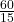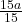Question

A race car starts from rest and speeds up uniformly to the right until it reaches a maximum velocity of 60m/s^2

What is the acceleration of the race car?

1.Thunguyet

Question:

A race car starts from rest and speed up uniformly to the right until it reaches a maximum velocity of 60m/s in 15 secs

4m/s²

Explanation:

The acceleration of a body in uniform motion can be found by using one of the equations of motion as follows;

v = u + at         ——————(i)

Where;

v = final velocity of the body

u = initial velocity of the body

a = acceleration of the body

t = time taken for the motion

From the question, the body is the race car and has the following:

v = 60m/s²

u = 0 [since the car starts from rest]

t = 15 secs.

Now, substitute these values into equation(i) as follows;

60 = 0 + a(15)

60 = 15a    [Divide through by 15]=a = 4

Therefore, the acceleration of the race car is 4m/s²